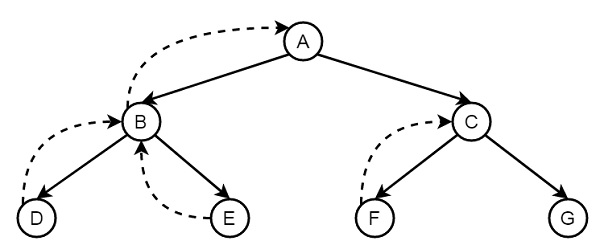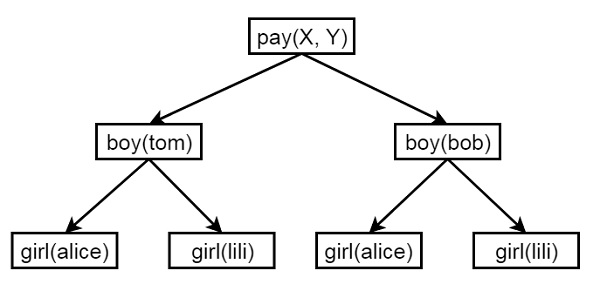# Prolog - Backtracking

In this chapter, we will discuss the backtracking in Prolog. Backtracking is a procedure, in which prolog searches the truth value of different predicates by checking whether they are correct or not. The backtracking term is quite common in algorithm designing, and in different programming environments. In Prolog, until it reaches proper destination, it tries to backtrack. When the destination is found, it stops.

Let us see how backtracking takes place using one tree like structure −Suppose A to G are some rules and facts. We start from A and want to reach G. The proper path will be A-C-G, but at first, it will go from A to B, then B to D. When it finds that D is not the destination, it backtracks to B, then go to E, and backtracks again to B, as there is no other child of B, then it backtracks to A, thus it searches for G, and finally found G in the path A-C-G. (Dashed lines are indicating the backtracking.) So when it finds G, it stops.

## How Backtracking works?

Now we know, what is the backtracking in Prolog. Let us see one example,

Note − While we are running some prolog code, during backtracking there may be multiple answers, we can press semicolon (;) to get next answers one by one, that helps to backtrack. Otherwise when we get one result, it will stop.

Now, consider a situation, where two people X and Y can pay each other, but the condition is that a boy can pay to a girl, so X will be a boy, and Y will be a girl. So for these we have defined some facts and rules −

### Knowledge Base

```boy(tom).
boy(bob).
girl(alice).
girl(lili).

pay(X,Y) :- boy(X), girl(Y).
```

Following is the illustration of the above scenario −As X will be a boy, so there are two choices, and for each boy there are two choices alice and lili. Now let us see the output, how backtracking is working.

### Output

```| ?- [backtrack].
compiling D:/TP Prolog/Sample_Codes/backtrack.pl for byte code...
D:/TP Prolog/Sample_Codes/backtrack.pl compiled, 5 lines read - 703 bytes written, 22 ms

yes
| ?- pay(X,Y).

X = tom
Y = alice ?

(15 ms) yes
| ?- pay(X,Y).

X = tom
Y = alice ? ;

X = tom
Y = lili ? ;

X = bob
Y = alice ? ;

X = bob
Y = lili

yes
| ?- trace.
The debugger will first creep -- showing everything (trace)

(16 ms) yes
{trace}
| ?- pay(X,Y).
1 1 Call: pay(_23,_24) ?
2 2 Call: boy(_23) ?
2 2 Exit: boy(tom) ?
3 2 Call: girl(_24) ?
3 2 Exit: girl(alice) ?
1 1 Exit: pay(tom,alice) ?

X = tom
Y = alice ? ;
1 1 Redo: pay(tom,alice) ?
3 2 Redo: girl(alice) ?
3 2 Exit: girl(lili) ?
1 1 Exit: pay(tom,lili) ?

X = tom
Y = lili ? ;
1 1 Redo: pay(tom,lili) ?
2 2 Redo: boy(tom) ?
2 2 Exit: boy(bob) ?
3 2 Call: girl(_24) ?
3 2 Exit: girl(alice) ?
1 1 Exit: pay(bob,alice) ?

X = bob
Y = alice ? ;
1 1 Redo: pay(bob,alice) ?
3 2 Redo: girl(alice) ?
3 2 Exit: girl(lili) ?
1 1 Exit: pay(bob,lili) ?
X = bob
Y = lili

yes
{trace}
| ?-
```

## Preventing Backtracking

So far we have seen some concepts of backtracking. Now let us see some drawbacks of backtracking. Sometimes we write the same predicates more than once when our program demands, for example to write recursive rules or to make some decision making systems. In such cases uncontrolled backtracking may cause inefficiency in a program. To resolve this, we will use the Cut in Prolog.

Suppose we have some rules as follows −

### Double step function

• Rule 1 &minnus; if X < 3 then Y = 0

• Rule 2 &minnus; if 3 <= X and X < 6 then Y = 2

• Rule 3 &minnus; if 6 <= X then Y = 4

In Prolog syntax we can write,

• f(X,0) :- X < 3. % Rule 1

• f(X,2) :- 3 =< X, X < 6. % Rule 2

• f(X,4) :- 6 =< X. % Rule 3

Now if we ask for a question as f (1,Y), 2 < Y.

The first goal f(1,Y) instantiated Y to 0. The second goal becomes 2 < 0 which fails. Prolog tries through backtracking two unfruitful alternatives (Rule 2 and Rule 3). If we see closer, we can observe that −

• The three rules are mutually exclusive and one of them at most will succeed.

• As soon as one of them succeeds there is no point in trying to use the others as they are bound to fail.

So we can use cut to resolve this. The cut can be expressed using Exclamation symbol. The prolog syntax is as follows −

• f (X,0) :- X < 3, !. % Rule 1

• f (X,2) :- 3 =< X, X < 6, !. % Rule 2

• f (X,4) :- 6 =< X. % Rule 3

Now if we use the same question, ?- f (1,Y), 2 < Y. Prolog choose rule 1 since 1 < 3 and fails the goal 2 < Y fails. Prolog will try to backtrack, but not beyond the point marked ! In the program, rule 2 and rule 3 will not be generated.

Let us see this in below execution −

### Program

```f(X,0) :- X < 3.              % Rule 1
f(X,2) :- 3 =< X, X < 6.   % Rule 2
f(X,4) :- 6 =< X.             % Rule 3
```

### Output

```| ?- [backtrack].
compiling D:/TP Prolog/Sample_Codes/backtrack.pl for byte code...
D:/TP Prolog/Sample_Codes/backtrack.pl compiled, 10 lines read - 1224 bytes written, 17 ms

yes
| ?- f(1,Y), 2<Y.

no
| ?- trace
.
The debugger will first creep -- showing everything (trace)

yes
{trace}
| ?- f(1,Y), 2<Y.
1 1 Call: f(1,_23) ?
2 2 Call: 1<3 ?
2 2 Exit: 1<3 ?
1 1 Exit: f(1,0) ?
3 1 Call: 2<0 ?
3 1 Fail: 2<0 ?
1 1 Redo: f(1,0) ?
2 2 Call: 3=<1 ?
2 2 Fail: 3=<1 ?
2 2 Call: 6=<1 ?
2 2 Fail: 6=<1 ?
1 1 Fail: f(1,_23) ?

(46 ms) no
{trace}
| ?-
```

Let us see the same using cut.

### Program

```f(X,0) :- X < 3,!.            % Rule 1
f(X,2) :- 3 =< X, X < 6,!. % Rule 2
f(X,4) :- 6 =< X.             % Rule 3
```

### Output

```| ?- [backtrack].
1 1 Call: [backtrack] ?
compiling D:/TP Prolog/Sample_Codes/backtrack.pl for byte code...
D:/TP Prolog/Sample_Codes/backtrack.pl compiled, 10 lines read - 1373 bytes written, 15 ms
1 1 Exit: [backtrack] ?
(16 ms) yes
{trace}
| ?- f(1,Y), 2<Y.
1 1 Call: f(1,_23) ?
2 2 Call: 1<3 ?
2 2 Exit: 1<3 ?
1 1 Exit: f(1,0) ?
3 1 Call: 2<0 ?
3 1 Fail: 2<0 ?
no
{trace}
| ?-
```

## Negation as Failure

Here we will perform failure when condition does not satisfy. Suppose we have a statement, “Mary likes all animals but snakes”, we will express this in Prolog.

It would be very easy and straight forward, if the statement is “Mary likes all animals”. In that case we can write “Mary likes X if X is an animal”. And in prolog we can write this statement as, likes(mary, X) := animal(X).

Our actual statement can be expressed as −

• If X is snake, then “Mary likes X” is not true

• Otherwise if X is an animal, then Mary likes X.

In prolog we can write this as −

• likes(mary,X) :- snake(X), !, fail.

• likes(mary, X) :- animal(X).

The ‘fail’ statement causes the failure. Now let us see how it works in Prolog.

### Program

```animal(dog).
animal(cat).
animal(elephant).
animal(tiger).
animal(cobra).
animal(python).

snake(cobra).
snake(python).

likes(mary, X) :- snake(X), !, fail.
likes(mary, X) :- animal(X).
```

### Output

```| ?- [negate_fail].
compiling D:/TP Prolog/Sample_Codes/negate_fail.pl for byte code...
D:/TP Prolog/Sample_Codes/negate_fail.pl compiled, 11 lines read - 1118 bytes written, 17 ms

yes
| ?- likes(mary,elephant).

yes
| ?- likes(mary,tiger).

yes
| ?- likes(mary,python).

no
| ?- likes(mary,cobra).

no
| ?- trace
.
The debugger will first creep -- showing everything (trace)

yes
{trace}
| ?- likes(mary,dog).
1 1 Call: likes(mary,dog) ?
2 2 Call: snake(dog) ?
2 2 Fail: snake(dog) ?
2 2 Call: animal(dog) ?
2 2 Exit: animal(dog) ?
1 1 Exit: likes(mary,dog) ?

yes
{trace}
| ?- likes(mary,python).
1 1 Call: likes(mary,python) ?
2 2 Call: snake(python) ?
2 2 Exit: snake(python) ?
3 2 Call: fail ?
3 2 Fail: fail ?
1 1 Fail: likes(mary,python) ?

no
{trace}
| ?-
```